# A Particle is in Linear Simple Harmonic Motion Between Two Points, a and B, 10 Cm Apart. Take the Direction from a to B as the Positive Direction and Give the Signs of Velocity, Acceleration and Force on the Particle When It is - Physics

A particle is in linear simple harmonic motion between two points, A and B, 10 cm apart. Take the direction from A to B as the positive direction and give the signs of velocity, acceleration and force on the particle when it is

(a) at the end A,

(b) at the end B,

(c) at the mid-point of AB going towards A,

(d) at 2 cm away from B going towards A,

(e) at 3 cm away from A going towards B, and

(f) at 4 cm away from B going towards A.

#### Solution 1

(a) Zero, Positive, Positive

(b) Zero, Negative, Negative

(c) Negative, Zero, Zero

(d) Negative, Negative, Negative

(e) Zero, Positive, Positive

(f) Negative, Negative, Negative

Explanation:

The given situation is shown in the following figure. Points A and B are the two end points, with AB = 10 cm. O is the midpoint of the path.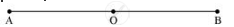A particle is in linear simple harmonic motion between the end points

a) At the extreme point A, the particle is at rest momentarily. Hence, its velocity is zero at this point.

Its acceleration is positive as it is directed along AO.

Force is also positive in this case as the particle is directed rightward.

b) At the extreme point B, the particle is at rest momentarily. Hence, its velocity is zero at this point.

Its acceleration is negative as it is directed along B.

Force is also negative in this case as the particle is directed leftward.

c)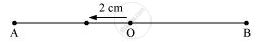The particle is executing a simple harmonic motion. O is the mean position of the particle. Its velocity at the mean position O is the maximum. The value for velocity is negative as the particle is directed leftward. The acceleration and force of a particle executing SHM is zero at the mean position.

d)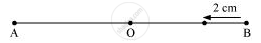The particle is moving toward point O from the end B. This direction of motion is opposite to the conventional positive direction, which is from A to B. Hence, the particle’s velocity and acceleration, and the force on it are all negative.

e)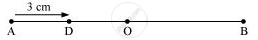The particle is moving toward point O from the end A. This direction of motion is from A to B, which is the conventional positive direction. Hence, the values for velocity, acceleration, and force are all positive.

f)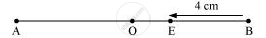This case is similar to the one given in (d).

#### Solution 2

In the fig. (given below), the points A and B, 10 cm apart, are the extreme positions of the particle in SHM, and the point O is the mean position. The direction from A to B is positive, as indicated.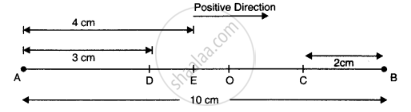a) At the end A, i.e., extreme position, velocity is zero, acceleration and force are directed towards O and are positive.

(b) At the end B, i.e., second extreme position, velocity is zero whereas the acceleration and force are directed towards the point O and are negative.

(c) At the mid point O, while going towards A, velocity is negative and maximum. The acceleration and force both are zero.

(d) At 2 cm away from B, that is, at C and going towards A: v is negative; acceleration and F, being directed towards O, are also negative.

(e) At 3 cm away from A, that is, at D and going towards B: v is positive; acceleration and F, being directed towards O, are also positive.

(f)  At a distance of 4 cm away from A and going towards A, velocity is directed along BA, therefore, it is positive. Since acceleration and force are directed towards OB, both of them are positive

Concept: Energy in Simple Harmonic Motion
Is there an error in this question or solution?

#### APPEARS IN

NCERT Class 11 Physics
Chapter 14 Oscillations
Q 5 | Page 358